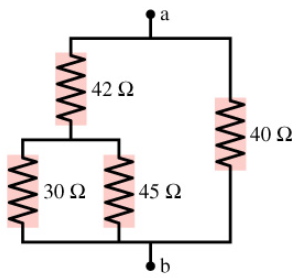# Problem: What is the equivalent resistance between points a and b in the figure? (Figure 1) Please show work.

🤓 Based on our data, we think this question is relevant for Professor MacDonald's class at ASU.

###### FREE Expert Solution

For resistors in parallel,

$\overline{)\frac{\mathbf{1}}{{\mathbf{R}}_{\mathbf{eff}}}{\mathbf{=}}\frac{\mathbf{1}}{{\mathbf{R}}_{\mathbf{1}}}{\mathbf{+}}\frac{\mathbf{1}}{{\mathbf{R}}_{\mathbf{2}}}{\mathbf{+}}\frac{\mathbf{1}}{{\mathbf{R}}_{\mathbf{3}}}{\mathbf{.}}{\mathbf{.}}{\mathbf{.}}}$

For resistors in series,

$\overline{){{\mathbf{R}}}_{\mathbf{e}\mathbf{f}\mathbf{f}}{\mathbf{=}}{{\mathbf{R}}}_{{\mathbf{1}}}{\mathbf{+}}{{\mathbf{R}}}_{{\mathbf{2}}}{\mathbf{+}}{{\mathbf{R}}}_{{\mathbf{3}}}{\mathbf{+}}{\mathbf{.}}{\mathbf{.}}{\mathbf{.}}}$###### Problem DetailsWhat is the equivalent resistance between points a and b in the figure? (Figure 1) Please show work.